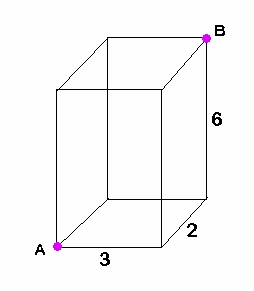# Slope

This week’s topic is:  Distance, Midpoint, and Slope.

### Q:  The slope of the line passing through (-1,-3) and (7,y) is -1/2.  What is the value of y?

.

.

.

.

Algebraic solution by hand:

Use the slope formula:Solution using my TI-84 Plus program DISTANCE:

Run DISTANCEHow?

Use your multiple choice answer choices.  One of them is -7.  (If you don’t have choices, draw a picture.  Plot the point (-1,-3) and do a slope of -1/2 from there – down 1, right 2, down 1, right 2, … until you get to a point where x is 7.  Read the y-value of that point – it will be -7 and check it using this program.)

x1=-1

y1=-3

x2=7

y2=-7

Ignore the distance information – we don’t need it for this problem.  Press ENTER for the midpoint information, which we don’t need either.  Press ENTER once more to find that the slope is -1/2.Lorem ipsum dolor sit amet, consectetur adipisicing elit, sed do eiusmod tempor incididunt ut labore et dolore magna aliqua. Ut enim ad minim veniam, quis nostrud exercitation ullamco laboris nisi ut aliquip ex ea commodo consequat.

# Distance

This week’s topic is:  Distance, Midpoint, and Slope.

### Q:  For what value(s) of x is the point (x,4) exactly 5 units away from the point (6,8)?

.

.

.

.

Solution by hand:

Use the distance formula:Solution using my TI-84 Plus program DISTANCE:

Draw a picture:Guess that x=2, maybe.  It’s probably an integer if this is an SAT problem.  Use the answer choices if available.

Run DISTANCEHow?

x1=2

y1=4

x2=6

y2=8

The distance is 5.66.  It’s too big – you wanted it to be 5 – so move the point a little closer to the middle.

Maybe x=3:

x1=3

y1=4

x2=6

y2=8

The distance is exactly 5.  Perfect.  (There’s also another possible answer, x=9.  If you have multiple choice answers, you will see 3 and 9 as a choice, so try both and see that they both work.  For a grid-in SAT problem, you would only have to find one answer anyway.)           :Lorem ipsum dolor sit amet, consectetur adipisicing elit, sed do eiusmod tempor incididunt ut labore et dolore magna aliqua. Ut enim ad minim veniam, quis nostrud exercitation ullamco laboris nisi ut aliquip ex ea commodo consequat.

# ACT, Geometry – diagonal of a rectangular prism

Q: What is the (straight-line) distance between points A and B on the figure below?Explanation:  We are finding the length of the green segment below:This requires the three-dimensional version of the distance formula:

There is another way to do this, using a progression of two right triangles, with the green segment being the hypotenuse of the second triangle, but I find the above formula much simpler.  It is just an extension of the ‘usual’ (2-dimensional) distance formula we are so familiar with from Algebra and Geometry.

BUT if you want an even easier way, and I know you do, use my TI-84 Plus program ‘DIST3D’.